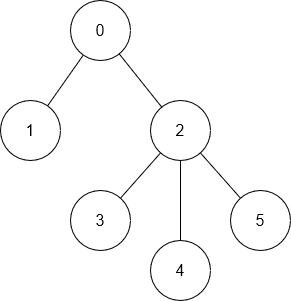# Longest Path in Tree

Posted: 5 Jul, 2021
Difficulty: Moderate

## PROBLEM STATEMENT

#### The longest path in a tree is defined as the maximum number of edges between any two nodes. You are given a 2-dimensional array ‘Edges’ in which ‘Edges[i]’ and ‘Edges[i]’ contains an edge.

##### For Example:
``````In the example below, the longest path is between nodes 1 and 3. The length of the path is 3 since the number of edges between node 1 and node 3 is 3. Hence, the answer is 3.
``````##### Input Format:
``````The first line of input contains an integer ‘T’ representing the number of test cases.

The first line of each test case contains an integer ‘N’ representing the number of nodes in the tree.

The following ‘N’ - 1 line of each test case contains two space-separated integers, ‘Edges[i]’ and ‘Edges[i]’ representing an edge between the two nodes.
``````
##### Output Format:
``````For each test case, print a single integer, the length of the longest path in the tree.

Print the output of each test case in a separate line.
``````
##### Note:
``````You do not need to print anything. It has already been taken care of. Just implement the function.
``````
##### Constraints:
``````1 <=  T <= 5
2 <= N <= 10^5
0 <= Edges[i], Edges[i] < N

Time limit: 1 sec
``````Approach 1

In this approach, we find the diameter of the tree recursively using Depth First Search. The diameter of the tree rooted at the current node is either:

• Diameter of the any of children node (If the current node is not part of the diameter)
• Sum of the height of two children + 1(current node is part of the diameter )

The diameter will be the maximum of these two values.

We will try to keep track of maximum and second maximum height among the children node at each node and find the maximum diameter among the children node. The diameter of the current node is the maximum of the sum of the two largest heights and the maximum diameter of children. To implement this, we will create a recursive function dfs(node, parent, tree), where the node is the current node, the parent is the parent node, and the tree is the adjacency list.

Algorithm:

• Make a function dfs(node, parent, tree): This returns an array containing the tree’s diameter rooted at node, and height of the node.
• Initialize two variables maxHeight and secondMaxHeight. This will denote the maximum and second maximum height of the child of the node.
• Set the variables currentHeight and currentDiameter as 0. The variable currentHeight and currentDiameter store the height and diameter of the current node.
• Iterate child through tree[node] and call dfs(child, node, tree) and get the childDiameter and childHeight.
• Keep track of the maximum and second maximum height of children in maxHeight and secondMaxHeight, respectively.
• maxChildDiameter is the maximum value of all childDiameter.
• Set currentHeight to maxHeight + 1.
• Value of currentDiameter is the maximum of maxHeight + secondMaxHeight and maxChildDiamter
• Return the list containing two integers, i.e., currentDiameter, and currentHeight from the function.
• Make an adjacency list tree from the Edges.
• Set answer as dfs(node, parent, tree).
• Return answer, i.e., the longest path of the tree.